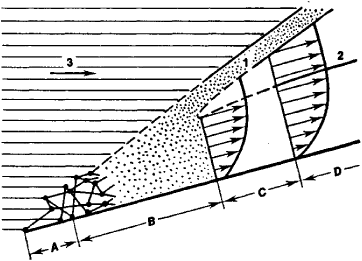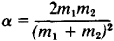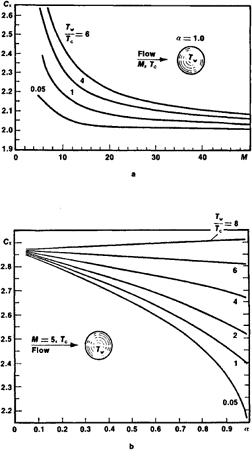# Aerodynamics of Rarefied Gases

The following article is from The Great Soviet Encyclopedia (1979). It might be outdated or ideologically biased.

## Aerodynamics of Rarefied Gases

a branch of the mechanics of gases in which their molecular structure must be taken into account in describing their motion. The methods of the aerodynamics of rarefied gases are widely applied in the determination of aerodynamic heating during the landing of orbital craft and for low-flying earth satellites, for the calculation of the thermal conditions of instrument transducers of rockets that are exploring the upper layers of the atmosphere, and so on. The exact prediction of the trajectories of low-altitude planetary satellites that are testing the braking action of rarefied atmosphere is impossible without knowledge of the methods of the aerodynamics of rarefied gases. Using these methods, it is possible to determine the aerodynamic forces and moments that act on an object moving in the gas. The aerodynamics of rarefied gases also investigates the flow of gases in vacuum systems, ultrasonic oscillations in gases, and other problems of molecular physics.

At high altitudes the atmosphere is very rarefied and the free path length l of molecules between two collisions becomes comparable with the characteristic dimension d of an object moving in the atmosphere (or the region of flow under consideration). Therefore, the methods of flow calculation that are used in aerodynamics and gas dynamics—based on the representation of a gas as a continuous medium (continuum)—are unsuitable, and it is necessary to resort to the kinetic theory of gases. At high gas temperatures—encountered, for example, at very high flight velocities—the flow can be accompanied by effects such as the excitation, dissociation, and ionization of molecules. These problems are also studied in the aerodynamics of rarefied gases.

The aerodynamics of rarefied gases is divided into three fields: (1) free molecular flow, (2) an intermediate region, and (3) slip flow (see Figure 1).Figure 1. Arbitrary schematic representation of various flows about a plane, long, infinitely thin plate around which a supersonic stream flows: (A) free molecular flow with single collisions, (8) intermediate region with multiple collisions, (C) slip flow, (D) continuum; (1) shock wave, (2) boundary of the boundary layer (arrows show the magnitude of the velocity at a given distance from the wall), (3) macroscopic motion of the molecules. (The scale of zones and regions is not true.)

Free molecular flow. In free molecular flow of molecules reflected from an object the free path length l is greater than the object’s characteristic dimension d, and so the interaction of the reflected molecules with the incident molecules near the object is insignificant. This makes it possible to consider the incident and reflected flow of molecules independently, which essentially facilitates the description of their motion. The motion of any molecule can be considered as consisting of two components: (1) the molecules take part in the directed motion of the gas flow and their velocity is equal to the aggregate flow velocity, (2) the molecules simultaneously take part in chaotic thermal motion and move with different velocities whose values are described by the Maxwell distribution. The application of the kinetic theory of gases makes possible the calculation of both the pressure of the gas on the wall and the amount of heat the wall gains or loses in interactions with gas molecules. In order to do this, it is necessary to know the laws of reflection of molecules from a hard surface.

However, the exact mathematical description of the motion of a rarefied gas by means of the equations of kinetic theory is rather difficult. Therefore, approximate methods have been developed. For example, the real reflection of molecules from an object is replaced by the so-called specular diffusion scheme, according to which part of the molecules are reflected from the object’s surface as from a mirror, and others are scattered diffusely in conformity with Lambert’s law (the cosine law).

The ratio of the number of diffusely scattered molecules to the total number determines the degree of diffusion of scattering, which is characterized by the number f (at f = 0, only mirrorlike reflection occurs; at f = 1, only diffuse reflection). In order to reduce the drag of a flying object, mirrorlike reflection is advantageous. Small angles of incidence of the molecules to the surface are also desirable because they increase the probability of mirrorlike reflection.

Another important parameter is the so-called thermal accommodation coefficient a, which characterizes the change in the molecule’s energy after its reflection. The values of α can vary from 0 to 1. If after reflection the energy of the molecule has not changed and remains equal to the energy of the incident molecule, then α = 0. If the average energy of the reflected molecule corresponds to the temperature of the wall, that means that the molecule has given up all its possible energy to the wall, and α = 1. It is obvious that aerodynamic heating decreases with decreasing α.

The quantities f and α are the most important characteristics of the aerodynamics of rarefied gases. In the general case, α and f depend on flow velocity of the gas, the material and temperature of the wall and the smoothness of its surface, the presence of adsorbed gas molecules on the surface, and so on. However, the exact dependences of α and f on the parameters determining them are still not known.

Experiments conducted over a wide velocity range with various gases and materials have yielded α values over a wide range—from 0.95 to 0.02. It has been established that a decrease in α occurs when the velocity of the gas molecules and the ratio of the molecular masses m, and m2 of the object and the gas are increased:Thus, for example, if a lead object is used instead of an aluminum one, the coefficient of accommodation is reduced by a factor of about 4, which leads to a reduction in aerodynamic heating. The coefficient / changes less—from 0.98 to 0.7.

The rarefaction of the medium becomes apparent in the rather unusual behavior of the aerodynamic coefficients. Thus, the drag coefficient of a sphere CD depends on the ratio of the absolute temperature of the object Tw to the absolute temperature of the flow Tt as well as on α and f (see Figure 2), and in a continuous medium such dependencies are not observed. The coefficients that characterize heat exchange also differ qualitatively and quantitatively from the continuous coefficients.Figure 2. Dependence of the resistance coefficient of the medium C, in a free molecular flow for different ratios of the absolute temperature of the body Tw to the absolute temperature of the flow Tc : (a) dependence on the Mach number (M) of the flow for α = 1.0; (b) dependence on the accommodation coefficient α.

Intermediate region. For l/d ∽ 1 the role of intermolecular collisions is significant when the molecules reflected from the object’s surface distort the velocity distribution of the molecules of the incident stream. The theoretical solution for free molecular flow is not suitable here. In addition, it is still not possible to consider such a flow as the flow of a continuous medium. The intermediate region is very difficult to analyze mathematically.

Slip flow. If the dimension of an object d is dozens of times larger than l—that is l/d≪1, then the shock waves and boundary layers at the surface of objects, which are characteristic of gas dynamics, can develop in the flow. However, in contrast to the ordinary boundary layer, the temperature Ta of the gas adjacent to the wall is not equal to the temperature of the wall Tw, and the flow velocity at the surface of the object is not 0 (the flow slips). The temperature jump (Tw - Ta) is proportional to l and depends on α. The slip velocity is also proportional to l and depends on f. Experiments have demonstrated that with the increase in rarefaction of the gas there is a thickening of the shock wave. The width of the boundary layer also increases, but considerably more slowly. The shock wave can propagate over the whole region of compressed gas in the region of the forward stagnation point of the streamlined object and combine with the boundary layer. The density distribution in the region of the forward stagnation point becomes smooth, not uneven as in a continuum. In the calculation of slip flows, the flow is described by the equations of gas dynamics, but with boundary conditions that take the temperature jump and the slip velocity into account.

The boundaries of the abovementioned regions of flow are very arbitrary. For different objects the appearance of the signs that characterize one or another region can occur at various values of the rarefaction parameter l\d. In connection with the complexity of theoretical calculations and the necessity of determining a number of empirical constants that are involved in practical methods of computation of thermal and aerodynamic characteristics, experimentation in the aerodynamics of rarefied gases is especially valuable.

### REFERENCES

Aerodinamika razrezhennykh gazov, collection 1. Edited by S. V. Vallander. Leningrad, 1963.
Patterson, G. N. Molekuliarnoe techeme gazov. Moscow, 1960. (Translated from English.)
Tsien, H. S. “Aerodinamika razrezhennykh gazov.” In Gazovaia dinamika (collection of articles). Edited by S. G. Popov and
S. V. Fal’kovich. Moscow, 1950. (Translated from English.)

L. V. KOZLOV